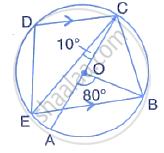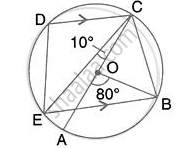Share

# In the Given Figure, Ac is the Diameter of the Circle with Centre O. Cd and Be Are Parallel. Angle ∠Aob = 80° and ∠Ace = 10°. Calculate: Angle Bec, - Mathematics

Course

#### Question

In the given figure, AC is the diameter of the circle with centre O. CD and BE are parallel. Angle ∠AOB = 80° and ∠ACE = 10°.

Calculate :  Angle BEC,#### Solution∠BOC = 180° - 80° = 100° (Straight line)

And ∠BOC = 2∠BEC

(Angle at the centre is double the angle at the circumference subtended by the same chord)

⇒ ∠BEC = (100°)/2 = 50°

Is there an error in this question or solution?